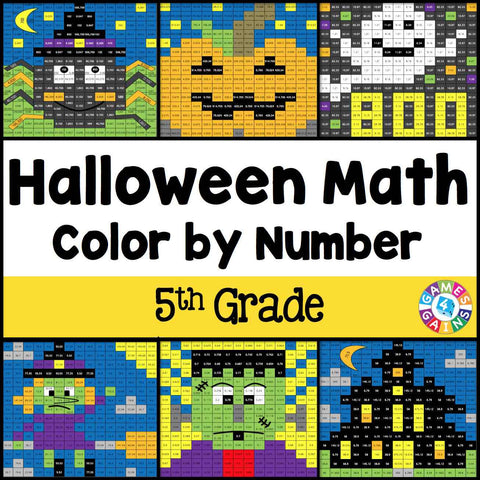## Halloween Math Color-by-Number - 5th Grade

• \$480

Halloween Math Color-by-Number set comes with 6 Halloween math color-by-number activities for reviewing 5th grade math skills. This Halloween math set is perfect to use in centers, in small groups, or with the whole class!

Included with this Halloween Math Color-by-Number set:
Instructions
6 color by number worksheets
6 student problem worksheets
6 teacher answer sheets

Here are the skills covered by these Halloween math color-by-number activities:

• Writing numbers with exponents in standard form
• Writing decimals
• Rounding decimals
• Comparing decimals
• Adding and subtracting decimals using the standard algorithm
• Adding and subtracting decimals in one-step and two-step word problems

Want to learn more about what you'll get? Check out the preview!